# A uniform thin rod of length 0.65 m and mass 3.4 kg can rotate in a horizontal plane about a vert... related homework questions

• #### A uniform thin rod of length 0.65 m and mass 3.4 kg can rotate in a horizontal plane about a vert...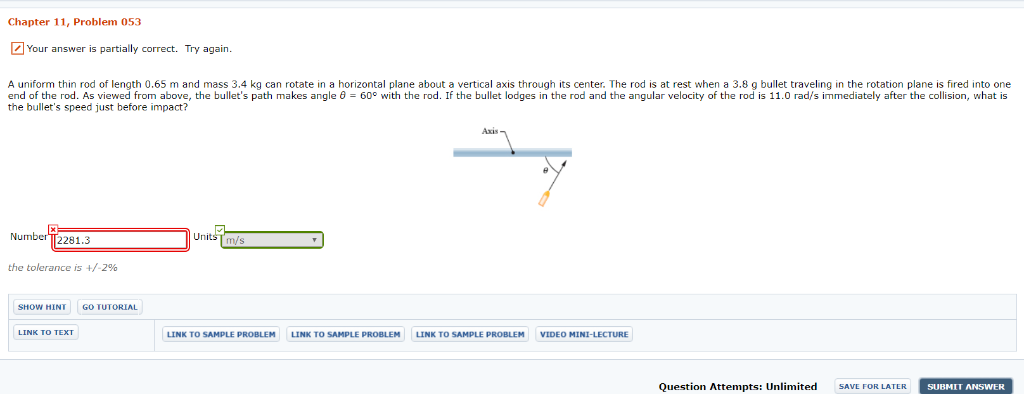A uniform thin rod of length 0.65 m and mass 3.4 kg can rotate in a horizontal plane about a vertical axis through its center. The rod is at rest when a 3.8 g bullet traveling in the rotation plane is fired into one end of the rod. As viewed from above, the bullet's path makes angle θ = 60° with the rod. If the bullet lodges in the rod and the angular ve...

• #### A slender 9 lb rod can rotate in a vertical plane about a pivot at B. A spring of constant k-30 lb/ft and of unstretched length 6 in. is attached to the rod as shown. The rod is released from rest in...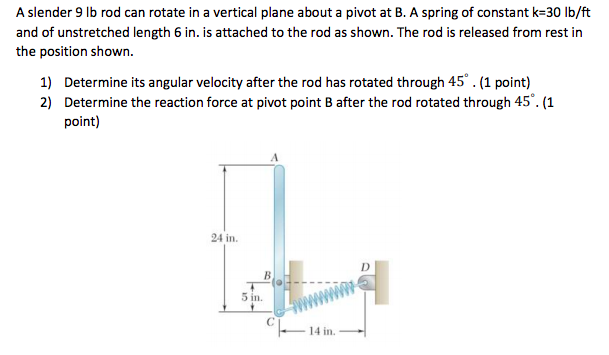A slender 9 lb rod can rotate in a vertical plane about a pivot at B. A spring of constant k-30 lb/ft and of unstretched length 6 in. is attached to the rod as shown. The rod is released from rest in the position shown. 1) Determine its angular velocity after the rod has rotated through 45.(1 point) 2) Determine the reaction force at pivot point B after the...

• #### Figure 3 Uniform disk Uniform rod 3) Figure 3 illustrates a physical pendulum comprising a uniform disk having mass M and radius R and a rod having the length R and mass M. The disk is pivotally m...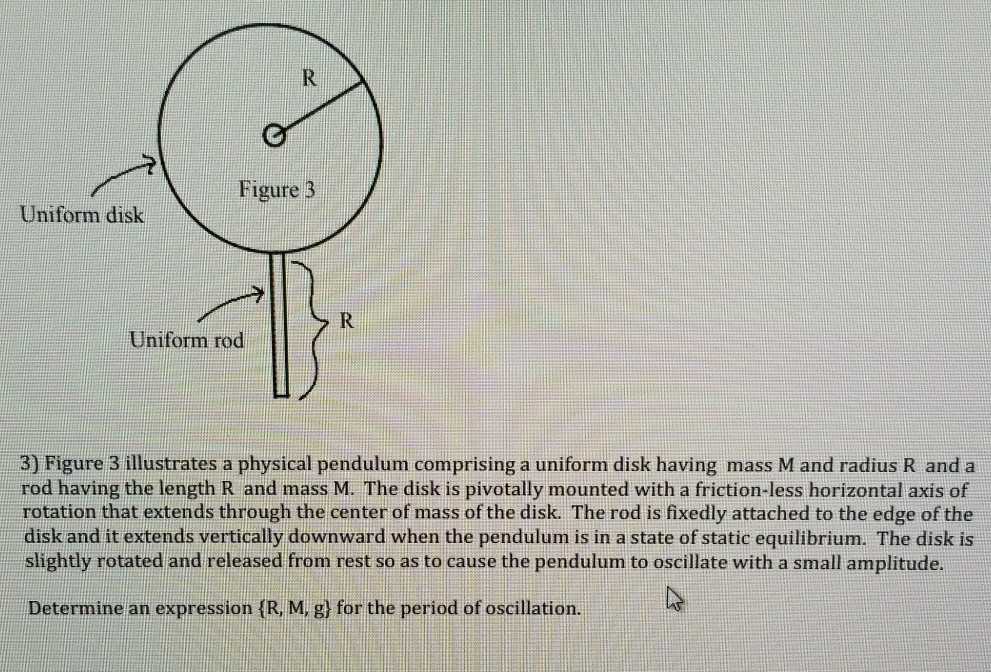Figure 3 Uniform disk Uniform rod 3) Figure 3 illustrates a physical pendulum comprising a uniform disk having mass M and radius R and a rod having the length R and mass M. The disk is pivotally mounted with a friction-less horizontal axis of rotation that extends through the center of mass of the disk. The rod is fixedly attached to the edge of the disk...

• #### Problem 2: The uniform rod of length, L, and mass, m, shown below, is released from rest and from an angle of 8- O (radians]. The rod then begins to rotate clockwise due to the force of gravity m...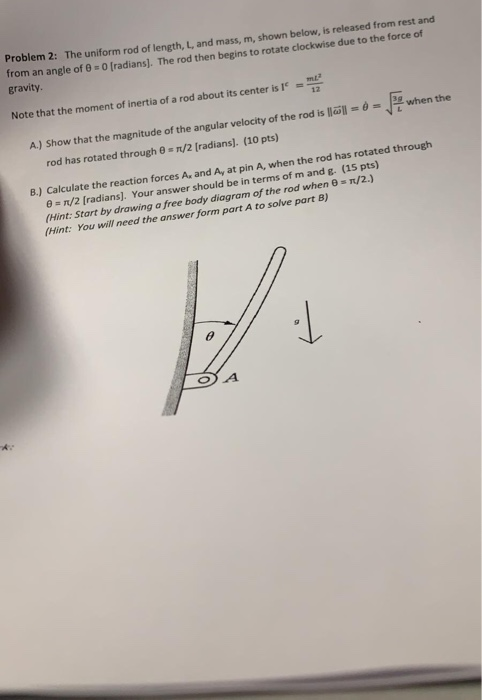Problem 2: The uniform rod of length, L, and mass, m, shown below, is released from rest and from an angle of 8- O (radians]. The rod then begins to rotate clockwise due to the force of gravity mE 12 Note that the moment of inertia of a rod about its center is when the A) Show that the magnitude of the angular velocity of the rod is löll- rod has rotate...

• #### These questions concern a space station, consisting of a long thin uniform rod of mass 4.3 x 10^6 kg and length 769 meters, with two identical uniform hollow spheres, each of mass 1.7 x 10^6 kg and ra...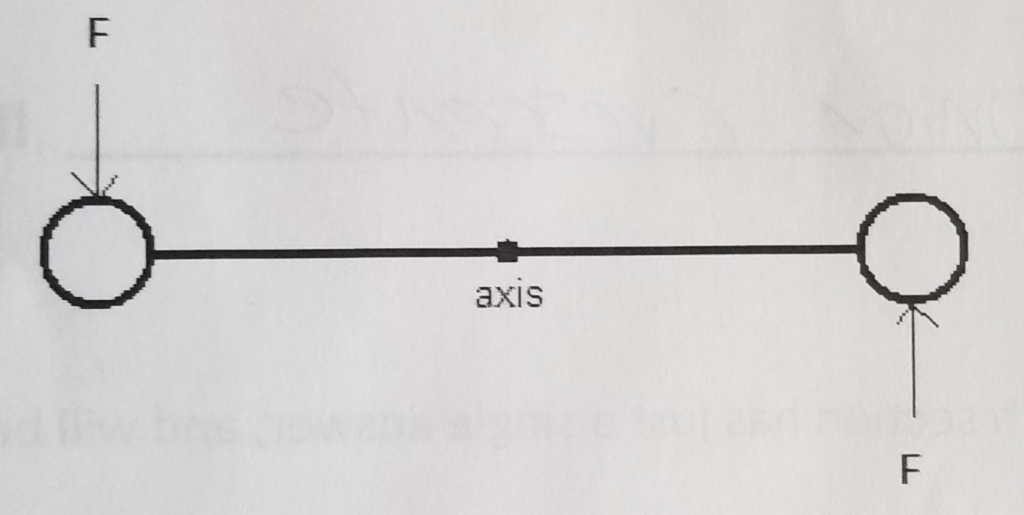These questions concern a space station, consisting of a long thin uniform rod of mass 4.3 x 10^6 kg and length 769 meters, with two identical uniform hollow spheres, each of mass 1.7 x 10^6 kg and radius 218 meters, attached at the ends of the rod, as shown below. Please note that none of the diagrams shown is drawn to scale. A. Suppose that the station st...

• #### M,R a = 0 14. A body consisted of an uniform rod of mass m and length L, and a disk of mass M and radius R can rotate a...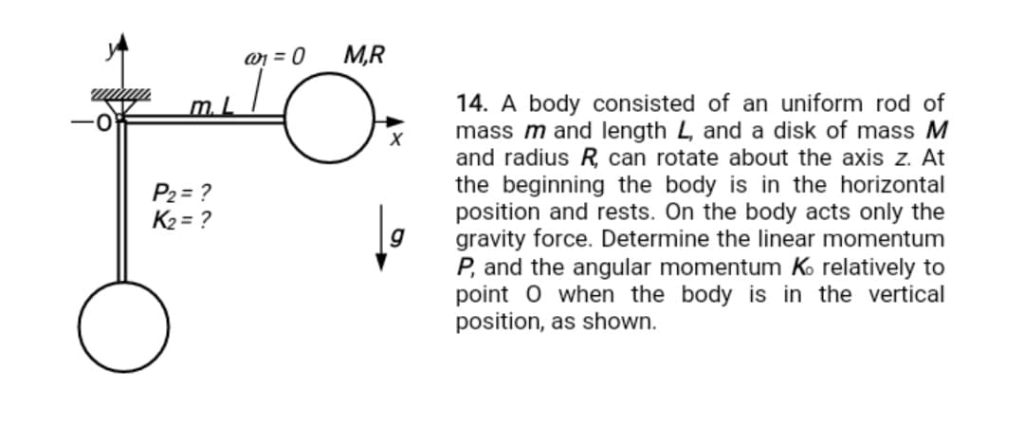M,R a = 0 14. A body consisted of an uniform rod of mass m and length L, and a disk of mass M and radius R can rotate about the axis z. At the beginning the body is in the horizontal position and rests. On the body acts only the gravity force. Determine the linear momentum P, and the angular momentum K relatively to point O when the body is in the vertical...

• #### All of the questions on this exam concern a space station, consisting of a long thin uniform rod of mass B ax10 kg and length C. 57meters, with two identical uniform hollow spheres, each of mass...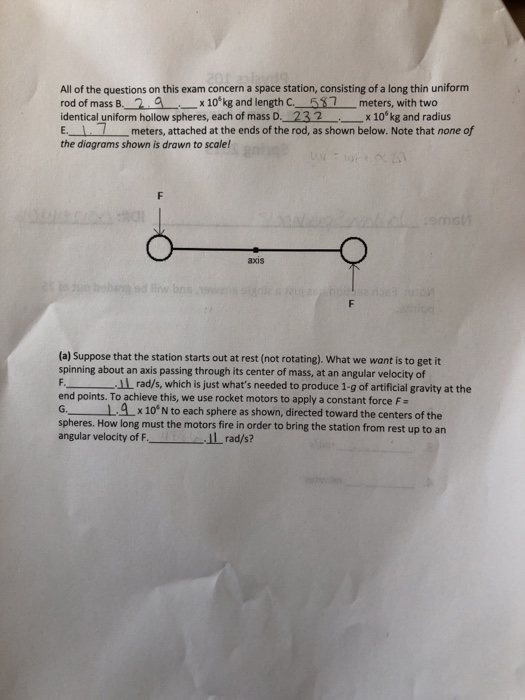All of the questions on this exam concern a space station, consisting of a long thin uniform rod of mass B ax10 kg and length C. 57meters, with two identical uniform hollow spheres, each of mass D._232 x10 kg and radius E. 1 meters, attached at the ends of the rod, as shown below. Note that none of the diagrams shown is drawn to scale! axis (a) Suppose...

• #### All of the questions on this concern a space station, consisting of a long thin uniform rod of mass 4.4 x 106kg and length 171 meters, with two identical uniform hollow spheres, each of mass 1.3 x 106...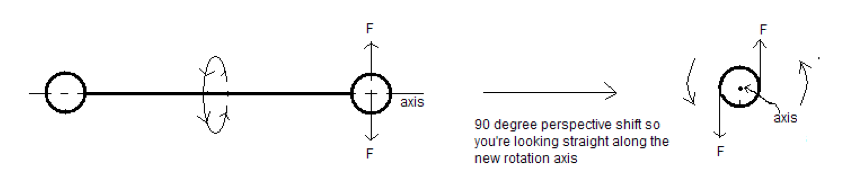All of the questions on this concern a space station, consisting of a long thin uniform rod of mass 4.4 x 106kg and length 171 meters, with two identical uniform hollow spheres, each of mass 1.3 x 106 kg and radius 57 meters, attached at the ends of the rod, as shown below. Note that none of the diagrams shown is drawn to scale! PART D: (d) Let's start...

• #### A thin rod, of length L and negligible mass, that can pivot about one end to rotate in a vertical circle

A thin rod, of length L and negligible mass, that can pivot about one end to rotate in a vertical circle. A heavy ball of mass m is attached to the other end. The rod is pulled aside through an angle and released. (a)What is the speed of the ball at the lowest point if L = 2.20 m, = 19.0°, and m = 500 kg? (b) Does the speed increase, decrease, or remain the...

• #### ANSWER ALL PARTS PLEASE long thin uniform All of the questions on this exam concern a space station, consisting of a rod of mass B._1.5__x 10°kg and length C. 23meters, with two identical uniform holl...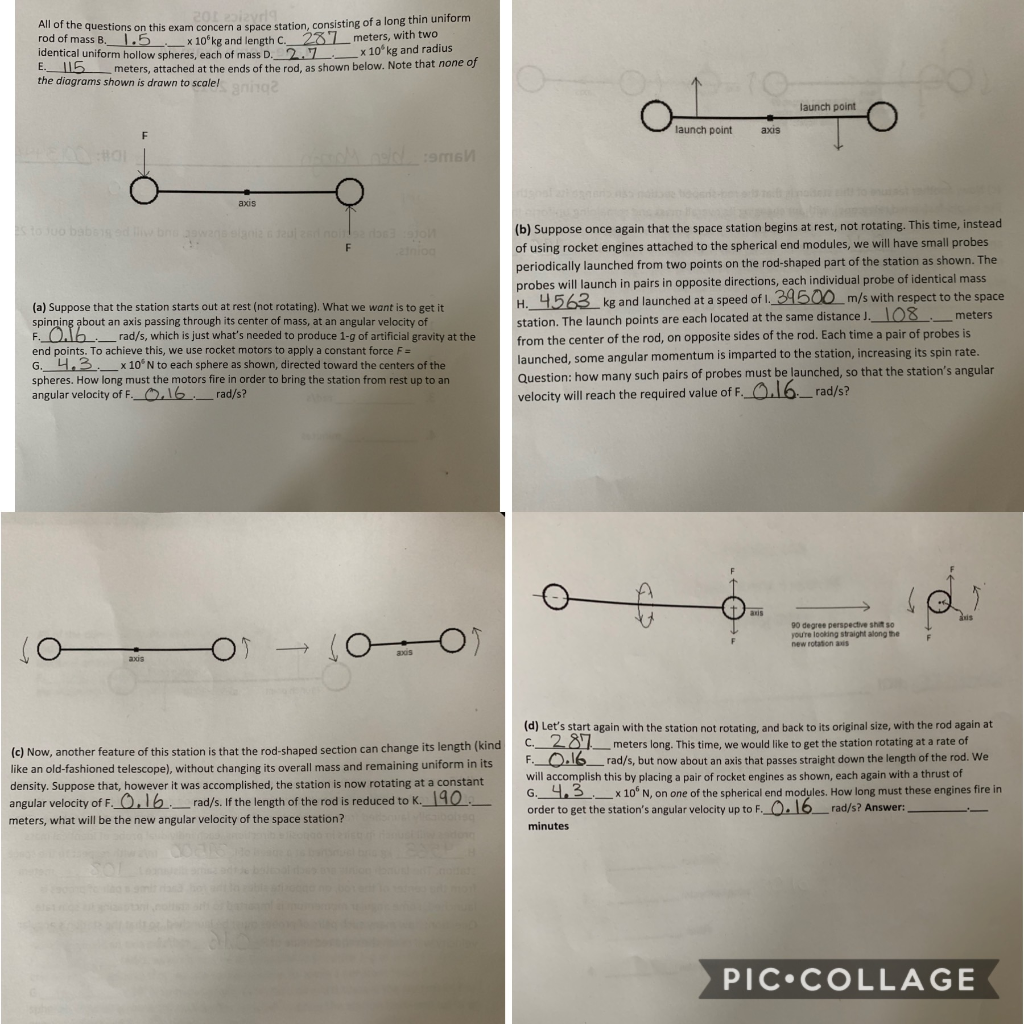ANSWER ALL PARTS PLEASE long thin uniform All of the questions on this exam concern a space station, consisting of a rod of mass B._1.5__x 10°kg and length C. 23meters, with two identical uniform hollow spheres, each of mass D.27x10' kg and radius tmeters, attached at the ends of the rod, as shown below. Note that none of the diagrams shown is drawn to s...

• #### What single rotation is equivalent to Rotate 40 degrees circle, Rotate 60 degrees circle, rotate -10 degrees

What single rotation is equivalent to Rotate 40 degrees circle, Rotate 60 degrees circle, rotate -10 degrees

• #### Pendulum A is a physical pendulum made from a thin, rigid, and uniform rod whose length is d. One end of this rod is at...

Pendulum A is a physical pendulum made from a thin, rigid, and uniform rod whose length is d. One end of this rod is attached to the ceiling by a frictionless hinge, so the rod is free to swing back and forth. Pendulum B is a simple pendulum whose length is also d. Obtain the ratio TA/TB of their periods for small-angle oscillations.

• #### Use the worked example above to help you solve this problem. A merry-go-round modeled as a disk of mass M 7.00 x 101 kg and radius R 2.40 m is rotating in a horizontal plane about a frictionless vert...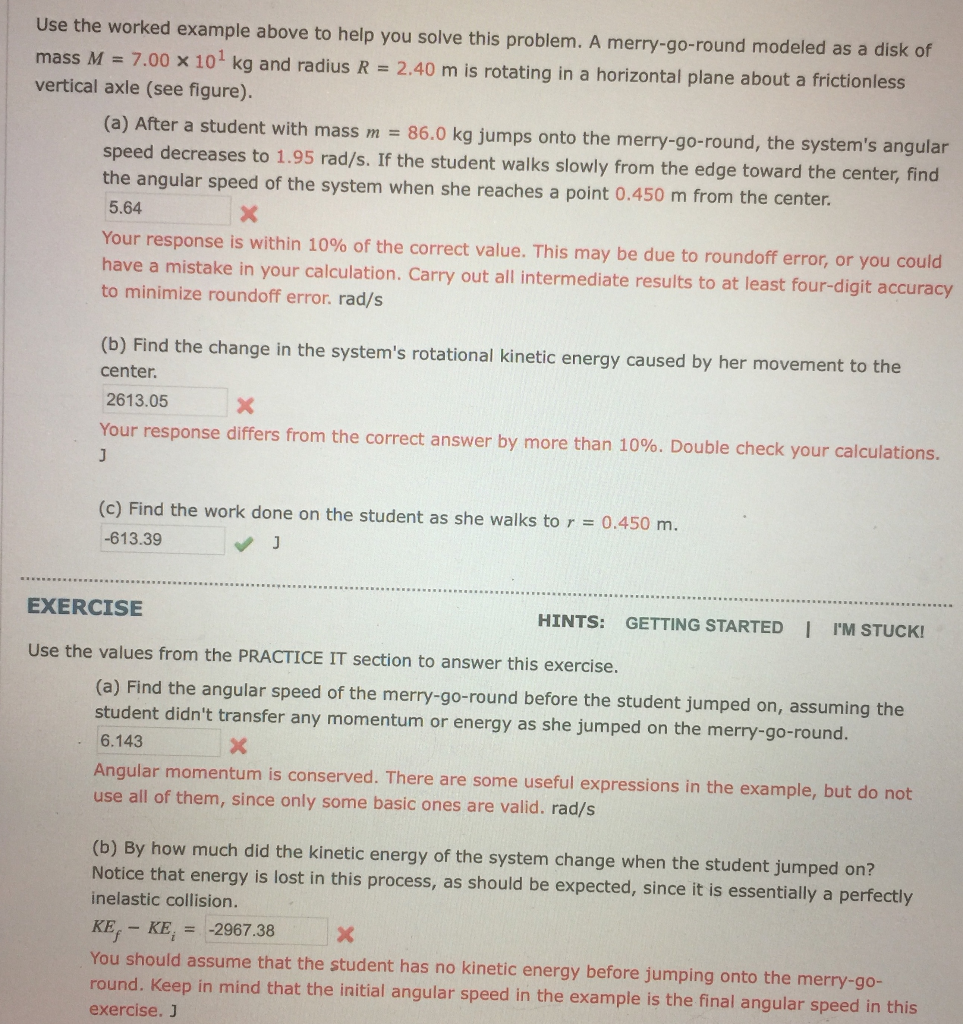Use the worked example above to help you solve this problem. A merry-go-round modeled as a disk of mass M 7.00 x 101 kg and radius R 2.40 m is rotating in a horizontal plane about a frictionless vertical axle (see figure). (a) After a student with mass m 86.0 kg jumps onto the merry-go-round, the system's angular speed decreases to 1.95 rad/s. If the st...

• #### An object with mass m is dragged along a horizontal plane by a force acting along a rope attached to the object as shown below mass horizontal plane If the rope makes an angle θ with the plane, then...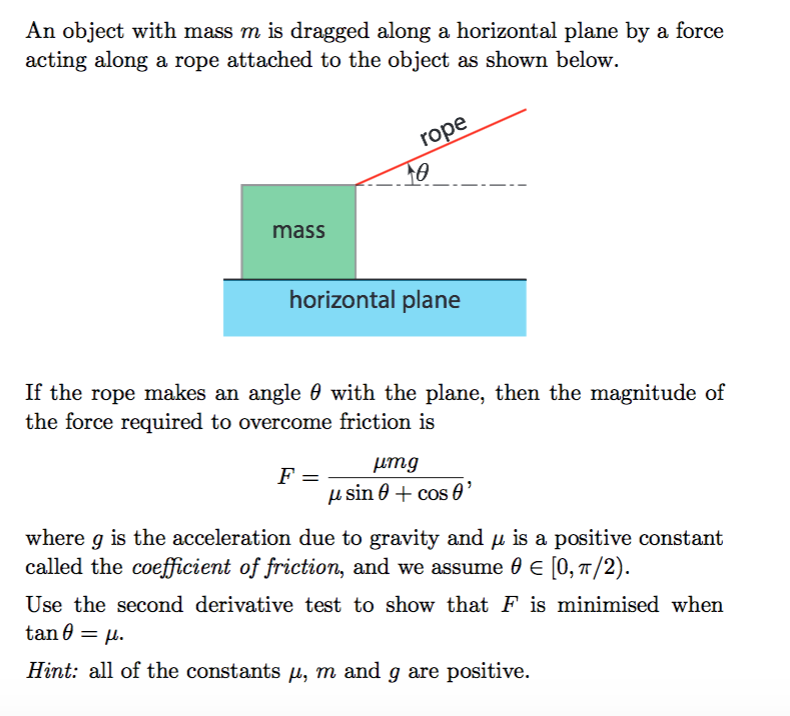An object with mass m is dragged along a horizontal plane by a force acting along a rope attached to the object as shown below mass horizontal plane If the rope makes an angle θ with the plane, then the magnitude of the force required to overcome friction is where g is the acceleration due to gravity and μ is a positive constant called the coefficient of ri...

• #### All of t rod of mass B. 2a _x10 kg and length C. 5meters, with two identical uniform hollow spheres, each of mass D. 232x 10 kg a E_ meters, attached at the ends of the rod, as shown below. Not...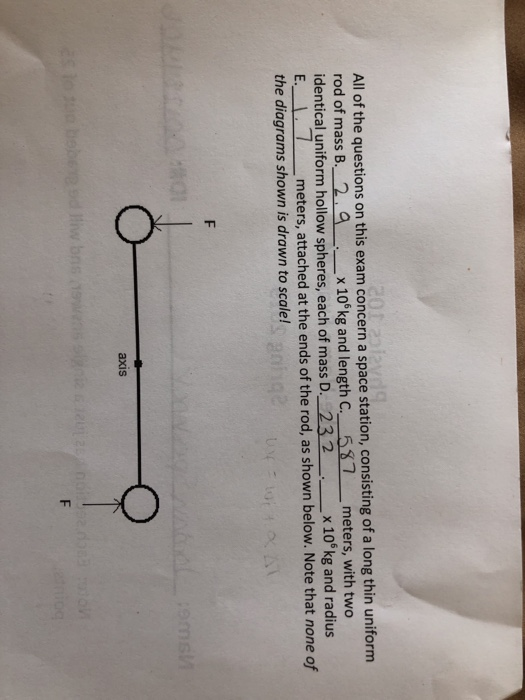All of t rod of mass B. 2a _x10 kg and length C. 5meters, with two identical uniform hollow spheres, each of mass D. 232x 10 kg a E_ meters, attached at the ends of the rod, as shown below. Note that none of the diagrams shown is drawn to scale! he questions on this exam concern a space station, consisting of a long thin uniform nd radius axis axis (e...

• #### Problen /) Derive equations of motion of the system shown below in x and 0 by using Lagrange's method. The thin rigid rod of length is supported as a pendulum at end A, and has a mass m. The rod...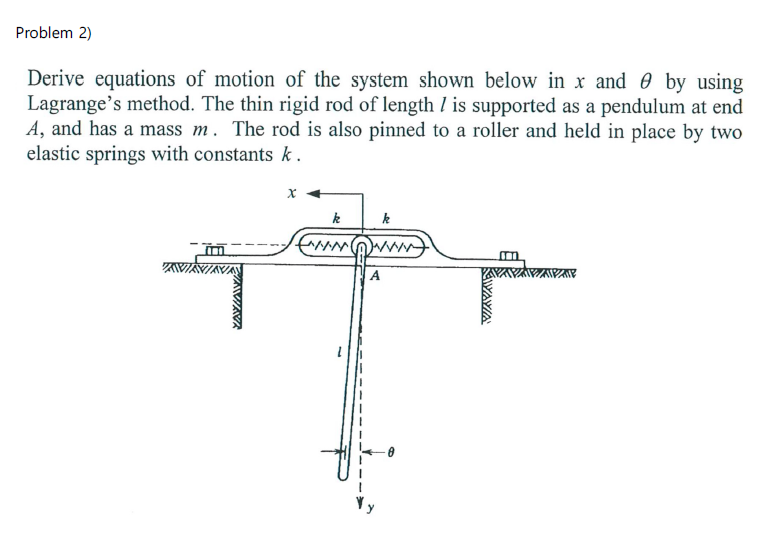Problen /) Derive equations of motion of the system shown below in x and 0 by using Lagrange's method. The thin rigid rod of length is supported as a pendulum at end A, and has a mass m. The rod is also pinned to a roller and held in place by two elastic springs with constants k . Problen /) Derive equations of motion of the system shown below in x...

• #### 44. The system shown in Fig. P7 consists of a slider block of mass m2 and a uniform slender rod of mass m3, length 13, and mass moment of inertia about its center of mass J The slider block is connec...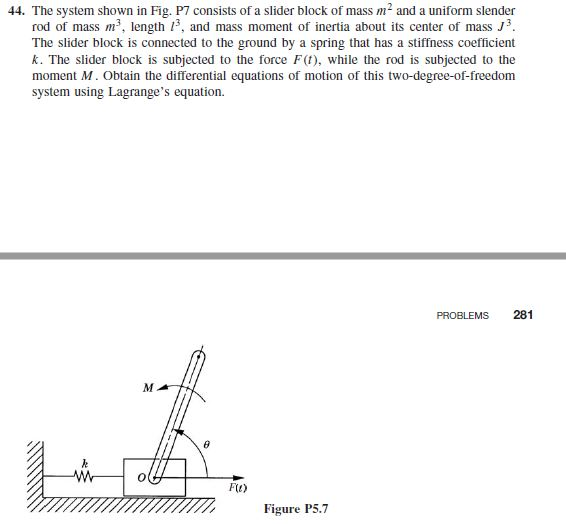44. The system shown in Fig. P7 consists of a slider block of mass m2 and a uniform slender rod of mass m3, length 13, and mass moment of inertia about its center of mass J The slider block is connected to the ground by a spring that has a stiffness coefficient k. The slider block is subjected to the force F(t), while the rod is subjected to the moment M. O...

• #### 3. A 2.8 cm x 5.0 cm rectangular rod is stretched from its initial length of 0.4000 m to a length of 0.4005 m. The Young’s modulus of the rod material is 95 GPa. Poisson’s ratio of the rod material is...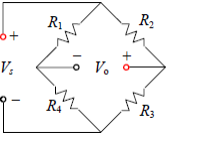3. A 2.8 cm x 5.0 cm rectangular rod is stretched from its initial length of 0.4000 m to a length of 0.4005 m. The Young’s modulus of the rod material is 95 GPa. Poisson’s ratio of the rod material is 0.333. A) Calculate the axial stress in units of MPa B) Calculate the transverse strain in units of microstrain C) A strain gauge with a strain gauge factor of...

• #### A) in minutes B) in launched pairs C) in rad/s All of the questions on this exam concern a space station, consisting of a long thin uniform rod of mass identical uniform hollow spheres, each o...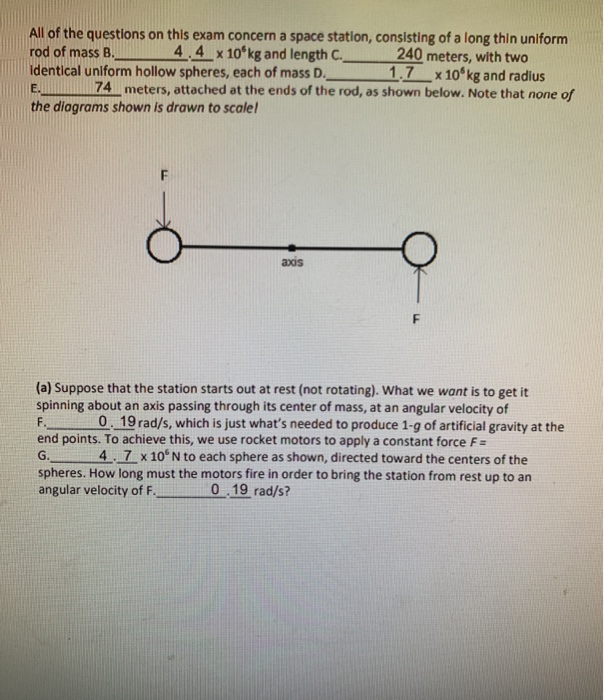A) in minutes B) in launched pairs C) in rad/s All of the questions on this exam concern a space station, consisting of a long thin uniform rod of mass identical uniform hollow spheres, each of mass D E 74-meters, attached at the ends of the rod, as shown below. Note that none of the diograms shown is drawn to scale 4 4x 10° kg and length C. 4.4 x10...

• #### please write clearly and and label ever answer All of the questions on this exam concern a space station, consisting of a long thin uniform rod of mass B. 니 x 106 kg and length C. D 3 meters, w...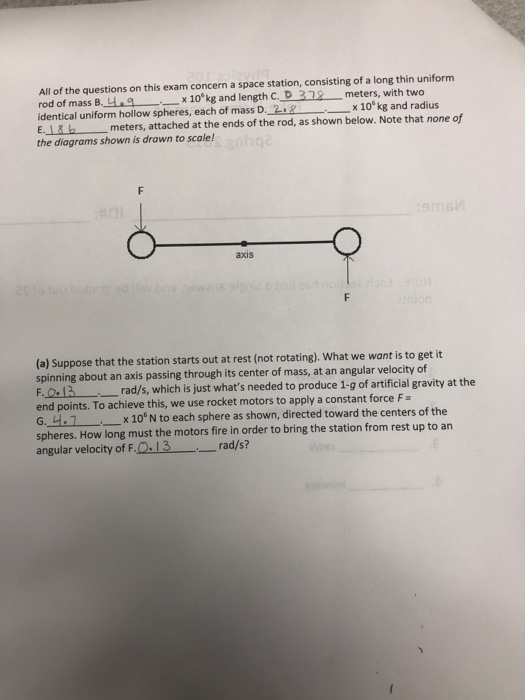please write clearly and and label ever answer All of the questions on this exam concern a space station, consisting of a long thin uniform rod of mass B. 니 x 106 kg and length C. D 3 meters, with two identical uniform hollow spheres, each of mass D. 2+2 x 10kg and radius Emeters, attached at the ends of the rod, as shown below. Note that none of the...

Need Online Homework Help?